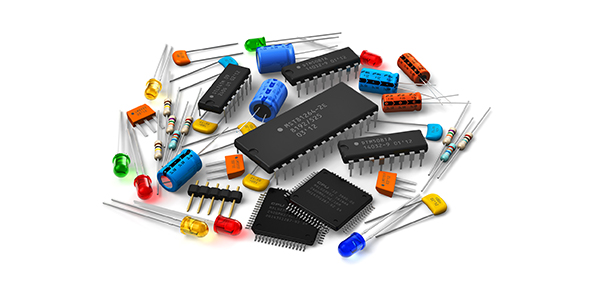# Electronics - Introduction To Semiconductor Theory

49 Questions | Total Attempts: 990SettingsThis includes semiconductor theory and diodes.

• 1.
Every known element has
• A.

The same type of atoms

• B.

the same number if atoms

• C.

A unique type of atom

• D.

Several different types of atoms

• 2.
An atom consists of
• A.

One nucleus and only one electron

• B.

One nucleus and one or more electrons

• C.

Protons, electrons, and neutrons

• D.

• 3.
The nucleus of an atom is made up of
• A.

Protons and neutrons

• B.

Electrons

• C.

Electrons and protons

• D.

Electrons and neutrons

• 4.
The atomic number of silicon is
• A.

8

• B.

2

• C.

4

• D.

14

• 5.
The atomic number of germanium is
• A.

8

• B.

2

• C.

4

• D.

32

• 6.
The valence shell in a silicon atom has the number designation of
• A.

0

• B.

1

• C.

2

• D.

3

• 7.
Valence electrons are
• A.

In the closest orbit to the nucleus

• B.

In the most distant orbit from the nucleus

• C.

In various orbits around the nucleus

• D.

Not associated with a particular atom

• 8.
A positive ion is formed when
• A.

A valence electron breaks away from the atom

• B.

there are more holes than electrons in the outer orbit

• C.

Two atoms bond together

• D.

An atom gains an extra valence electron

• 9.
The most widely used semiconductive material in electronic devices is
• A.

Germanium

• B.

Carbon

• C.

Copper

• D.

Silicon

• 10.
The energy band in which free electrons exist is the
• A.

First band

• B.

Second band

• C.

Conduction band

• D.

Valence band

• 11.
Electron-hole pairs are produced by
• A.

Recombination

• B.

Thermal energy

• C.

Ionization

• D.

Doping

• 12.
Recombination is when
• A.

An electron falls into a hole

• B.

A positive and a negative ion bond together

• C.

A valence electron becomes a conduction electron

• D.

A crystal is formed

• 13.
In a semiconductor crystal, the atoms are held together by
• A.

The interaction of valence electrons

• B.

Forces of attraction

• C.

Covalent bonds

• D.

• 14.
Each atom  in a silicon crystal has
• A.

Four valence electrons

• B.

Four conduction electrons

• C.

Eight valence electrons, four of its own and four shared

• D.

No valence electrons because all are shared with other atoms

• 15.
The current in a semiconductor is produced by
• A.

Electron only

• B.

Holes only

• C.

Negative ions

• D.

Both electrons and holes

• 16.
In an intrinsic semiconductor,
• A.

There are no free electrons

• B.

The free electrons are thermally produced

• C.

There are only holes

• D.

There are many electrons as there are holes

• E.

• 17.
The difference between an insulator and a semiconductor is
• A.

A wider energy gap between the valence band and the conduction band

• B.

The number of free electrons

• C.

The atomic structure

• D.

• 18.
The process of adding an impurity to an intrinsic semiconductor is called
• A.

Doping

• B.

Recombination

• C.

Atomic modification

• D.

Ionization

• 19.
The trivalent impurity is added to silicon to create
• A.

Germanium

• B.

A p-type semiconductor

• C.

An n-type semiconductor

• D.

A depletion region

• 20.
The purpose of pentavalent impurity is to
• A.

Reduce the conductivity of silicon

• B.

Increase the number of holes

• C.

Increase the number of free electrons

• D.

Create minority carriers

• 21.
The majority carriers in an n-type semiconductor are
• A.

Holes

• B.

Valence electrons

• C.

Conduction electrons

• D.

Protons

• 22.
Holes in an n-type semiconductor are
• A.

Minority carriers that are thermally produced

• B.

Minority carriers that are produced by doping

• C.

Majority carriers that are thermally produced

• D.

Majority carriers that are produced by doping

• 23.
A pn junction is formed by
• A.

The recombination of electrons and holes

• B.

Ionization

• C.

The boundary of a p-type and an n-type material

• D.

The collision of a proton and a neutron

• 24.
The depletion region is created by
• A.

Ionization

• B.

Diffusion

• C.

Recombinations

• D.

• 25.
The depletion region consists of
• A.

Nothing but minority carriers

• B.

Positive and negative ions

• C.

No majority carriers

• D.Back to top